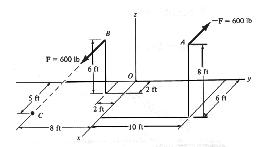# Problem: Determine the couple moment. Use a vector analysis and express the result as a Cartesian vector.

###### FREE Expert Solution

The moment about O due to applied force at B is:

$\overline{){{\mathbf{M}}}_{{\mathbf{O}}}{\mathbf{=}}{{\mathbf{r}}}_{\mathbf{O}\mathbf{B}}{\mathbf{×}}{\mathbf{F}}}$, where rOB is the position vector between B and O and F is the resultant force.

Cross product:

The resultant force is calculated by Certasian vector notation.

We resolve each force into x- and y-components the add the repetitive components by scalar algebra.

Resultant force:

$\overline{)\begin{array}{rcl}{\mathbf{F}}_{\mathbf{n}\mathbf{e}\mathbf{t}}& {\mathbf{=}}& \mathbf{\left(}{\mathbf{F}}_{\mathbf{1}\mathbf{x}}\mathbf{i}\mathbf{+}{\mathbf{F}}_{\mathbf{1}\mathbf{y}}\mathbf{j}\mathbf{\right)}\mathbf{+}\mathbf{\left(}{\mathbf{F}}_{\mathbf{2}\mathbf{x}}\mathbf{i}\mathbf{+}{\mathbf{F}}_{\mathbf{2}\mathbf{y}}\mathbf{j}\mathbf{\right)}\\ & {\mathbf{=}}& \mathbf{\left(}{\mathbf{F}}_{\mathbf{1}\mathbf{x}}\mathbf{+}{\mathbf{F}}_{\mathbf{2}\mathbf{x}}\mathbf{\right)}\mathbf{i}\mathbf{+}\mathbf{\left(}{\mathbf{F}}_{\mathbf{1}\mathbf{y}}\mathbf{+}{\mathbf{F}}_{\mathbf{2}\mathbf{y}}\mathbf{\right)}\mathbf{j}\\ & {\mathbf{=}}& \mathbf{\sum }{\mathbf{F}}_{\mathbf{x}}\mathbf{i}\mathbf{+}\mathbf{\sum }{\mathbf{F}}_{\mathbf{y}}\mathbf{j}\end{array}}$

The position vector between two points is given by:

$\overline{)\begin{array}{rcl}{\mathbf{r}}_{\mathbf{A}\mathbf{B}}& {\mathbf{=}}& \mathbf{\left(}{\mathbf{x}}_{\mathbf{B}}\mathbf{-}{\mathbf{x}}_{\mathbf{A}}\mathbf{\right)}\mathbf{i}\mathbf{+}\mathbf{\left(}{\mathbf{y}}_{\mathbf{B}}\mathbf{-}{\mathbf{y}}_{\mathbf{A}}\mathbf{\right)}\mathbf{j}\mathbf{+}\mathbf{\left(}{\mathbf{z}}_{\mathbf{B}}\mathbf{-}{\mathbf{z}}_{\mathbf{A}}\mathbf{\right)}\mathbf{k}\end{array}}$

We also need to know some general rules for vector cross products.

$\overline{)\begin{array}{rcl}\mathbf{i}\mathbf{×}\mathbf{i}& {\mathbf{=}}& {\mathbf{0}}\\ \mathbf{i}\mathbf{×}\mathbf{j}& {\mathbf{=}}& {\mathbf{k}}\\ \mathbf{i}\mathbf{×}\mathbf{k}& {\mathbf{=}}& \mathbf{-}\mathbf{j}\\ \mathbf{j}\mathbf{×}\mathbf{j}& {\mathbf{=}}& {\mathbf{0}}\\ \mathbf{j}\mathbf{×}\mathbf{i}& {\mathbf{=}}& \mathbf{-}\mathbf{k}\\ \mathbf{j}\mathbf{×}\mathbf{k}& {\mathbf{=}}& {\mathbf{i}}\\ \mathbf{k}\mathbf{×}\mathbf{k}& {\mathbf{=}}& {\mathbf{0}}\\ \mathbf{k}\mathbf{×}\mathbf{i}& {\mathbf{=}}& {\mathbf{j}}\\ \mathbf{k}\mathbf{×}\mathbf{j}& {\mathbf{=}}& \mathbf{-}\mathbf{i}\end{array}}$

89% (451 ratings)###### Problem DetailsDetermine the couple moment. Use a vector analysis and express the result as a Cartesian vector.

Frequently Asked Questions

What scientific concept do you need to know in order to solve this problem?

Our tutors have indicated that to solve this problem you will need to apply the Intro to Cross Product (Vector Product) concept. If you need more Intro to Cross Product (Vector Product) practice, you can also practice Intro to Cross Product (Vector Product) practice problems.